Function Repository Resource:

# SubspaceBasis

Find a basis for the subspace spanned by a list of vectors

Contributed by: Dennis M Schneider
 ResourceFunction["SubspaceBasis"][vecs] returns a basis for the subspace spanned by vecs. ResourceFunction["SubspaceBasis"][polys,x,"Polynomial"] returns a basis for the subspace spanned by a list of polynomials in x. ResourceFunction["SubspaceBasis"][funcs,x] returns a basis for the subspace spanned by a list of functions of x.

## Details and Options

The vecs argument should be a list of vectors in n or n, or a list of matrices whose elements are in n or n.
ResourceFunction["SubspaceBasis"][list] can be used to determine if the vectors in the list are independent when compared with the resource function LinearlyIndependent.
ResourceFunction["SubspaceBasis"][vecs,x] attempts to find a basis for the functions in the list by expressing the zero function as a linear combination of the functions and then generating a list of homogeneous equations obtained by substituting k = Length[vecs] random real numbers into the equation, thereby obtaining a square matrix. Then the members of the list corresponding to the pivot columns of the reduced echelon form of the matrix are selected to obtain the independent functions in the list.
When the input is a set of functions, ResourceFunction["SubspaceBasis"] returns a set that may be ill-conditioned.

## Examples

### Basic Examples (5)

A basis for the subspace spanned by a dependent list of vectors in 4:

 In:=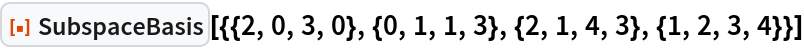Out=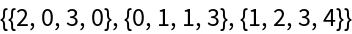If the vectors in the list are independent, then SubspaceBasis[list] returns a list:

 In:=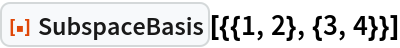Out=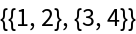Test whether a list of vectors is independent (compare with ResourceFunction["LinearlyIndependent"]):

 In:=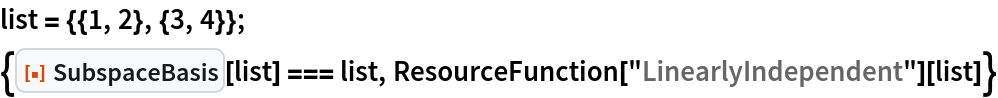Out=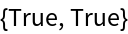A basis for the subspace spanned by a dependent list of three 2×2 matrices:

 In:=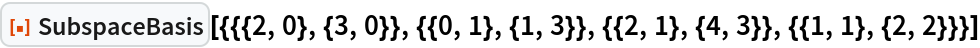Out=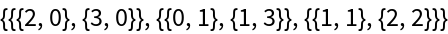The 2×2 symmetric matrices together with the 2×2 trace zero matrices do not form an independent list of matrices:

 In:=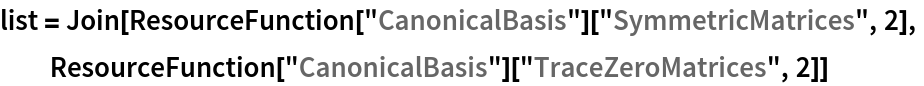Out=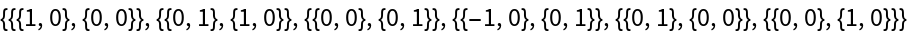In:=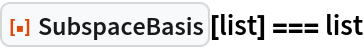Out=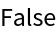A basis for the span of this list of matrices:

 In:=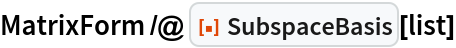Out=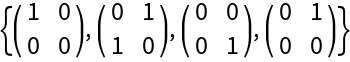A basis for the subspace spanned by a dependent list of polynomials in x:

 In:=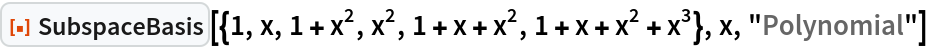Out=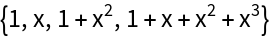A basis for the subspace spanned by a dependent list of trig functions:

 In:=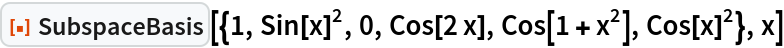Out=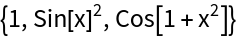Test that a list of trigonometric polynomials is linearly independent:

 In:=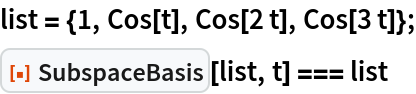Out=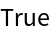### Possible Issues (2)

When the input is a set of functions, SubspaceBasis uses a method that may be ill-conditioned:

 In:=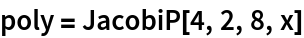Out=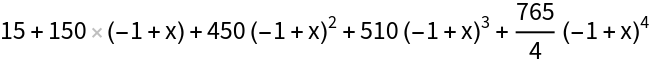In:=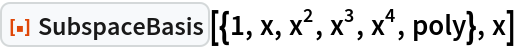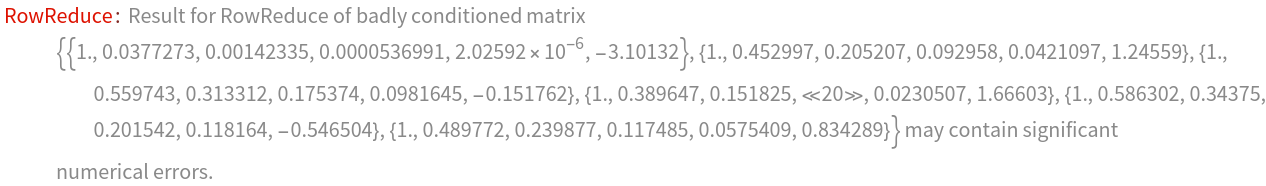Out=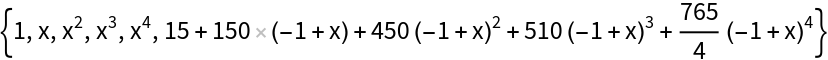Specifying polynomial inputs gives a better result:

 In:=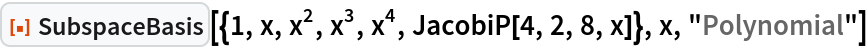Out=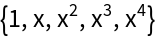## Publisher

Dennis M Schneider

## Version History

• 1.0.0 – 18 September 2020